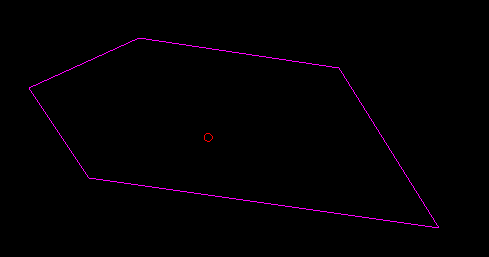# Rotate 2D polygon to _specified_ rotation

Say I have a 2D polygon with 5 sides which I’ve defined like this:

``````Vertices = new Vector2
{
new Vector2(140, 100),
new Vector2(250, 50),
new Vector2(450, 80),
new Vector2(550, 240),
new Vector2(200, 190)
}
``````

Which looks like this:With the centre of mass at the red circle. Assuming that the rotation of the polygon as it’s initialised is 0.0f radians and I want to rotate it around the COM to X radians… How do I do that?

What I have at the moment is this, where `position` is the desired position of the polygon on screen.

``````transformMatrix = Matrix.CreateTranslation(-Center.X + Position.X, -Center.Y + Position.Y, 0.0f) *
Matrix.CreateRotationZ(currentAngle) *
Matrix.CreateTranslation(Center.X - Position.X, Center.Y - Position.X, 0.0f);

for (int i = 0; i < Vertices.Length; i++)
{
Vertices[i] = Vector2.Transform(Vertices[i], transformMatrix);
}
``````

But I don’t want to treat `currentAngle` as a relative rotation (If I run this code in the update at the moment, it just spins in place with the angle incremented by `currentAngle`). I want to be able to just say

`currentAngle = MathHelper.ToRadians(degreesRotation);`

And just have the polygon pointing in that direction. Or do:

`currentAngle += 0.01f;`

in the update and have it spin place.

How do I go about achieving that?

EDIT:
Polygon class with this problem solved here:

I’m not sure if I’ve understood this correctly, but you want to have your polygon to go any specified rotation and not incremented, right? So you want to say, rotate to 45 degrees, and it does. Then say rotate to 90 degrees, and it does… instead of rotating to 135, which would be the sum of those two rotations?

If I’ve understood this correctly, I can think of two options…

1. Don’t update your original points with the new rotation. Instead, write a method that requests an array of rotated points while the original remains intact. This way you can request a rotation of whatever you want. This will probably give you the most accurate rotation, but you’ll increase your memory allocations (probably not a big deal). You could also just store two arrays in your polygon class… original vertices and transformed vertices.
2. Whenever you rotate, first rotate by the negative of the previous rotation, then rotate by the new rotation. So, if your points initialize to 0 degrees rotation, then you want to rotate by 45 degrees, rotate by negative 0 (ie, nothing) and then rotate by 45 degrees. Then, when you want to rotate by 90 degrees, rotate by -45 degrees, then rotate by 90 degrees. This keeps the vertex storage combined to a single array; however, there will likely be cascading small errors that will add up over time.

Personally I’d recommend the first approach, but it’s up to you. Those are just my ideas though… and all assuming I’ve understood what you want to do correctlyThat’s exactly what I ended up going with! Thanks!

Here’s my code in case anyone else wants to do this same thing:

``````class Polygon
{
private Vector2 Position;
private float currentAngle = 0.0f;

public Vector2[] WorldVertices;

/// <summary>
/// Create a polygon defined by <paramref name="vertices"/>, centered around <paramref name="position"/>.
/// </summary>
/// <param name="vertices">An array of Vector2 positions defining the vertices of the polygon.</param>
/// <param name="position">The position, in world space, around which the polygon should be centered.</param>
public Polygon(Vector2[] vertices, Vector2 position)
{
Position = position;
WorldVertices = new Vector2[vertices.Length];
ModelVertices = new Vector2[vertices.Length];

Vector2 center = CenterOfPolygon(vertices);

//Cache the model vertices positioned adjusted to be around the origin
for (int i = 0; i < vertices.Length; i++)
{
ModelVertices[i] = vertices[i] - center;
}

for (int i = 0; i < vertices.Length; i++)
{
WorldVertices[i] = vertices[i];
}
}

public void Update()
{
//Rotate the polygon by 3.5 degrees every frame
currentAngle += 3.5f;

//Use the ModelVertices offsets to calculate new positions for the WorldVertices to be rendered
//See https://en.wikipedia.org/wiki/Rotation_(mathematics)#Two_dimensions for the trig explanation
for (int i = 0; i < WorldVertices.Length; i++)
{
WorldVertices[i].X = Position.X +
ModelVertices[i].X * (float) Math.Cos(angle) +
ModelVertices[i].Y * (float) Math.Sin(angle);

WorldVertices[i].Y = Position.Y -
ModelVertices[i].X * (float) Math.Sin(angle) +
ModelVertices[i].Y * (float) Math.Cos(angle);
}

Position = Input.MousePosition();
}

/// <summary>
/// Calculates the center of a polygon defined by <paramref name="vertices"/>
/// </summary>
/// <param name="vertices">An array of Vector2 positions defining the vertices of the polygon.</param>
/// <returns>A Vector2 representing the world space position of the center of the given polygon.</returns>
public static Vector2 CenterOfPolygon(Vector2[] vertices)
{
Vector2 sumCentre = Vector2.Zero;
float sumWeight = 0.0f;

for (int i = 0; i < vertices.Length; i++)
{
int prevIndex = (i - 1 + vertices.Length) % vertices.Length;
int nextIndex = (i + 1) % vertices.Length;

float weight = Vector2.Distance(vertices[i], vertices[prevIndex]) +
Vector2.Distance(vertices[i], vertices[nextIndex]);

sumCentre += vertices[i] * weight;
sumWeight += weight;
}

return sumCentre / sumWeight;
}
}``````
1 Like

NiceOne suggestion is that you might consider only recalculating your vertices when the angle actually changes. I know right now you have it changing every time `Update` is called, but you can pull that up a level so that you can update the angle on the Polygon object itself, which then will update the vertices.

The only distinction here is that when the angle isn’t changing, you aren’t doing any actual work. Ditto for the position.

Yeah, you’re 100% correct. For me this is just for debugging polygon collisions in non-production code so it’s not a huge deal, but anyone wanting to use the above code for anything more important they should absolutely take that into account!1 Like

In general you should always keep the original polygon unmodified and perform just in time transforms. This is friendlier to video memory too, since vertices can be stored in read optimized memory. You also won’t accumulate floating point error that way, and I think it’s easier to avoid Euler lock.